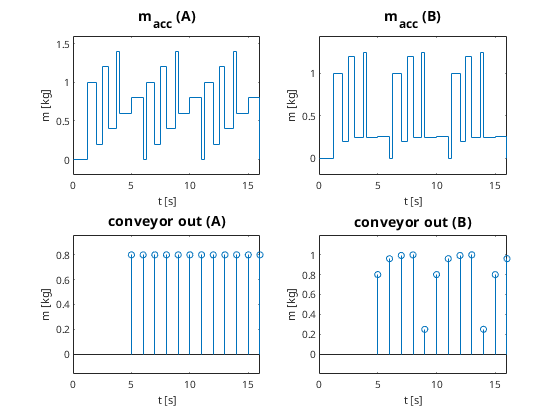### Coupling of conveyors with different velocities

• Problem:
• two coupled conveyors, lengths l1,2 and constant velocities v1,2
• continuous process for connected conveyors
•• → simply change line load λ2 by factor v1/v2
• discrete process with different lE1,2 and ∆t1,2
• conveyor 1 sends entities with time intervals ∆t1
• conveyor 2 outputs entities with time intervals ∆t2
• timing doesn't fit
•• Basic idea:
• entity ≙ content of a given compartment on a conveyor
• created and filled at the entrance
• emptied and destroyed at the exit
• how to compute mass mout of a new entity for conveyor 2?
• requirements
• mass conservation on short time scale
• homogeneity, i. e. output distribution similar to input
• crucial parameter
•• k > 1 → add up incoming masses to one compartment
• k < 1 → distribute incoming mass to several compartments
• simple case k∊ℕ → add up k incoming masses
• simple case 1/k∊ℕ → distribute incoming mass to 1/k outgoing masses
• Strategy for k > 1:
• introduce virtual bin with mass macc between conveyors
• incoming entities fill bin
• outgoing entity empties bin
• example
• lE1 = lE2 = 1 m, v1 = 2.5 m/s, v2 = 1 m/s, min ≡ 1 kg
• →  ∆t1 = 0.4 s, ∆t2 = 1 s, k = 2.5
•• Strategy A for k < 1:
• again virtual bin with mass macc between conveyors
• each time min arrives: compute partition mass mp
• mp = k min
• mass of outgoing entity
• mout = min(mp, macc)
• good example
• ∆t1 = 1 s, ∆t2 = 0.35 s → k = 0.35
•• very homogenous distribution
• ∆t1 = 1 s, ∆t2 = 0.8 s → k = 0.8
•• macc grows → short time mass conservation violated
• Strategy B for k < 1:
• like A, but changed partition size
• mp = k macc
• distribute the surplus of macc
• precise mathematical description in paper
• bad example (k = 0.8)
•• comparison
•• B: much better short time mass conservation
• A: better homogeneity